# Panel Methods What are panel methods Panel methods

• Slides: 19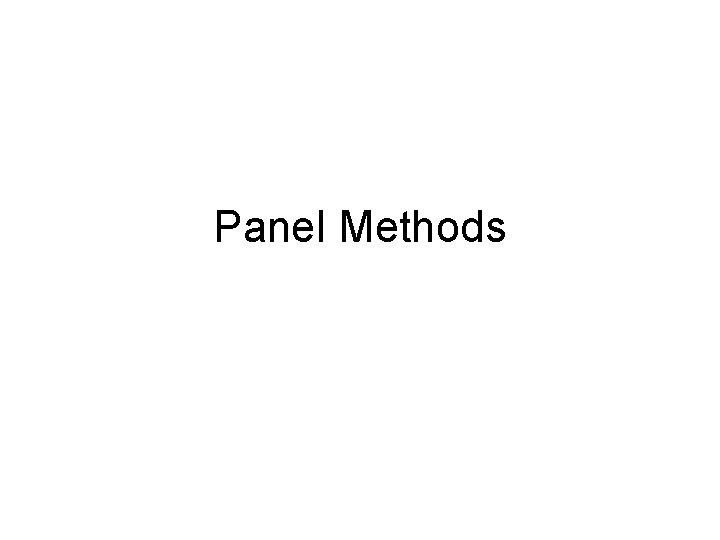Panel Methods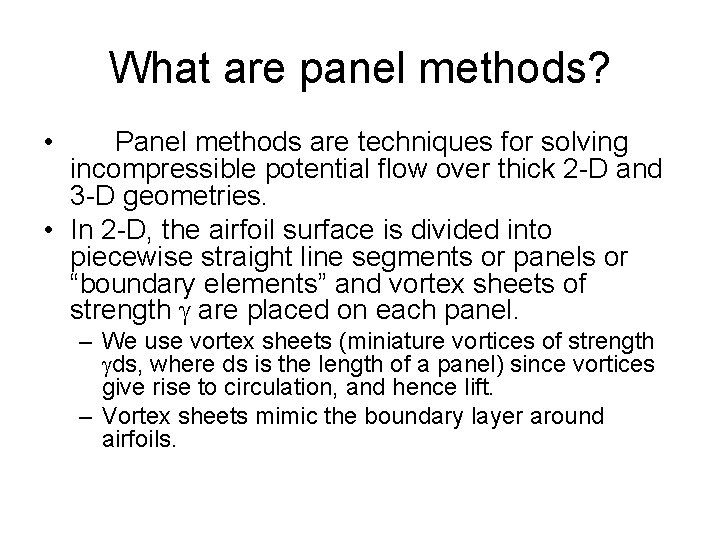What are panel methods? • Panel methods are techniques for solving incompressible potential flow over thick 2 -D and 3 -D geometries. • In 2 -D, the airfoil surface is divided into piecewise straight line segments or panels or “boundary elements” and vortex sheets of strength g are placed on each panel. – We use vortex sheets (miniature vortices of strength gds, where ds is the length of a panel) since vortices give rise to circulation, and hence lift. – Vortex sheets mimic the boundary layer around airfoils.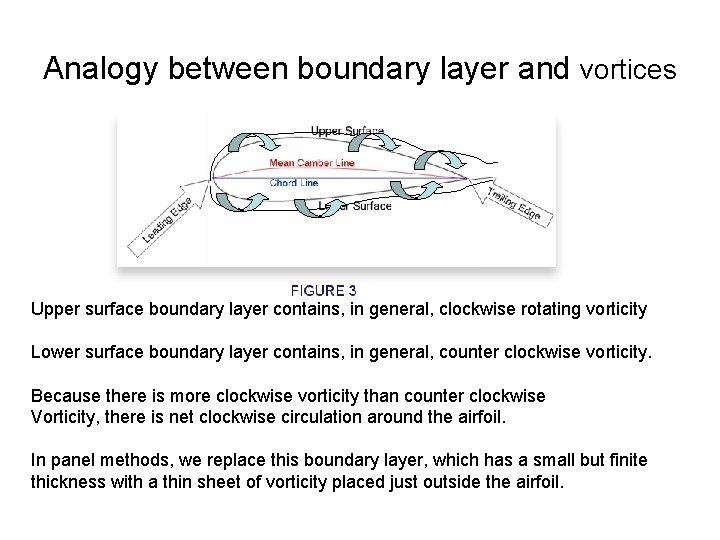Analogy between boundary layer and vortices Upper surface boundary layer contains, in general, clockwise rotating vorticity Lower surface boundary layer contains, in general, counter clockwise vorticity. Because there is more clockwise vorticity than counter clockwise Vorticity, there is net clockwise circulation around the airfoil. In panel methods, we replace this boundary layer, which has a small but finite thickness with a thin sheet of vorticity placed just outside the airfoil.Panel method treats the airfoil as a series of line segments On each panel, there is vortex sheet of strength DG = g 0 ds 0 Where ds 0 is the panel length. Each panel is defined by its two end points (panel joints) and by the control point, located at the panel center, where we will Apply the boundary condition y= Constant=C. The more the number of panels, the more accurate the solution, since we are representing a continuous curve by a series of broken straight lines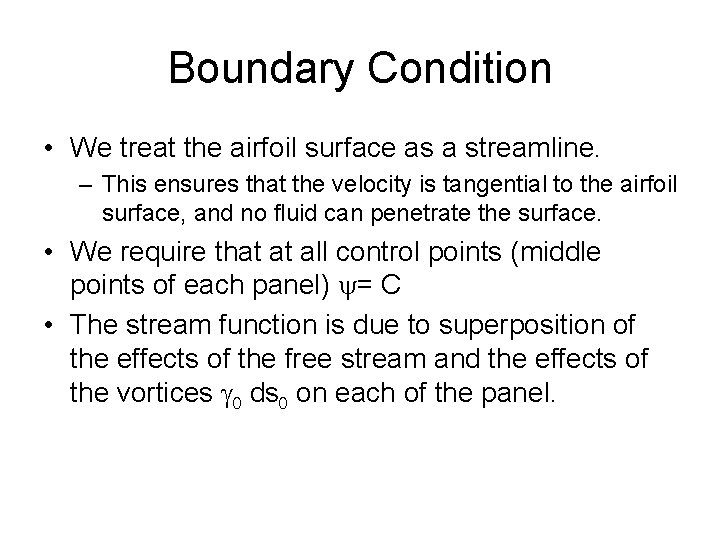Boundary Condition • We treat the airfoil surface as a streamline. – This ensures that the velocity is tangential to the airfoil surface, and no fluid can penetrate the surface. • We require that at all control points (middle points of each panel) y= C • The stream function is due to superposition of the effects of the free stream and the effects of the vortices g 0 ds 0 on each of the panel.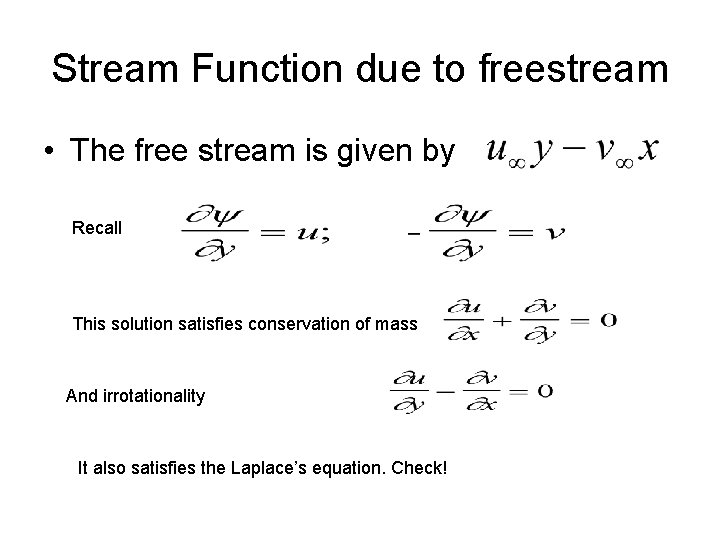Stream Function due to freestream • The free stream is given by Recall This solution satisfies conservation of mass And irrotationality It also satisfies the Laplace’s equation. Check!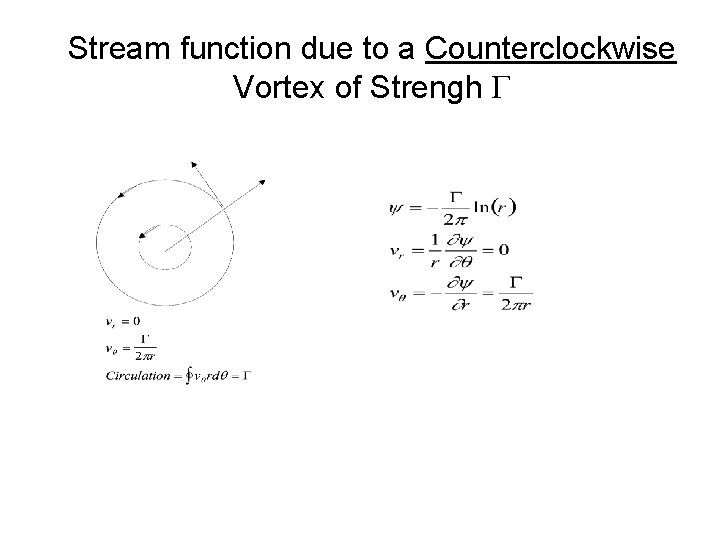Stream function due to a Counterclockwise Vortex of Strengh G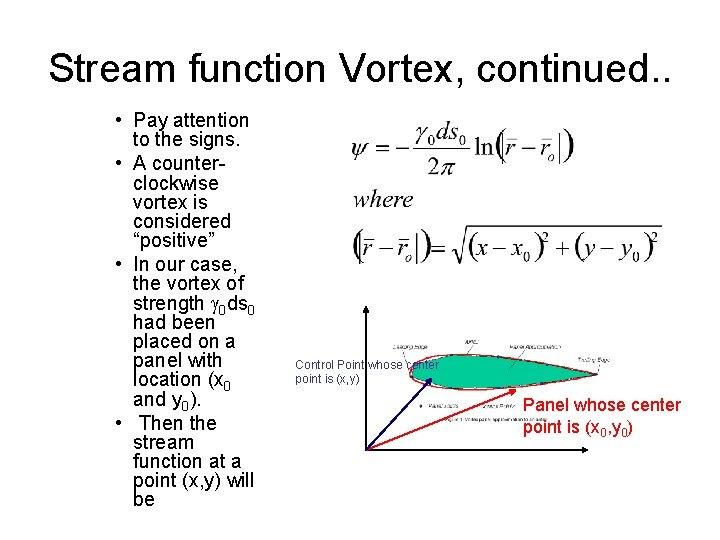Stream function Vortex, continued. . • Pay attention to the signs. • A counterclockwise vortex is considered “positive” • In our case, the vortex of strength g 0 ds 0 had been placed on a panel with location (x 0 and y 0). • Then the stream function at a point (x, y) will be Control Point whose center point is (x, y) Panel whose center point is (x 0, y 0)Superposition of All Vortices on all Panels • In the panel method we use here, ds 0 is the length of a small segment of the airfoil, and g 0 is the vortex strength per unit length. • Then, the stream function due to all such infinitesimal vortices at the control point (located in the middle of each panel) may be written as the interval below, where the integral is done over all the vortex elements on the airfoil surface.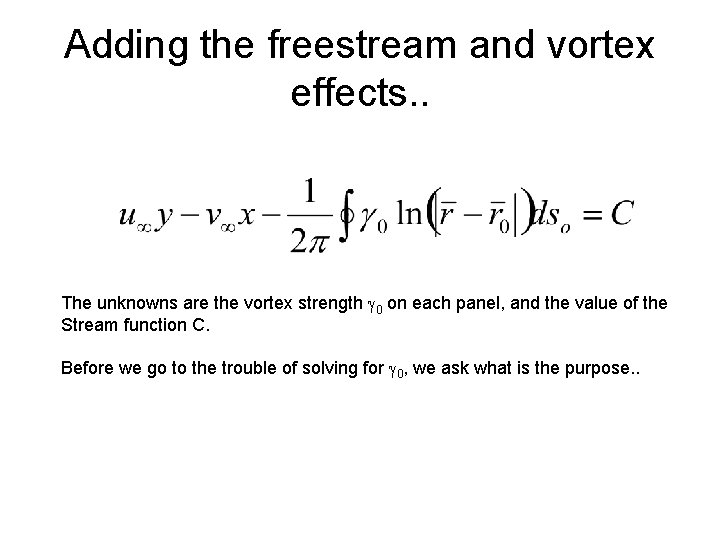Adding the freestream and vortex effects. . The unknowns are the vortex strength g 0 on each panel, and the value of the Stream function C. Before we go to the trouble of solving for g 0, we ask what is the purpose. .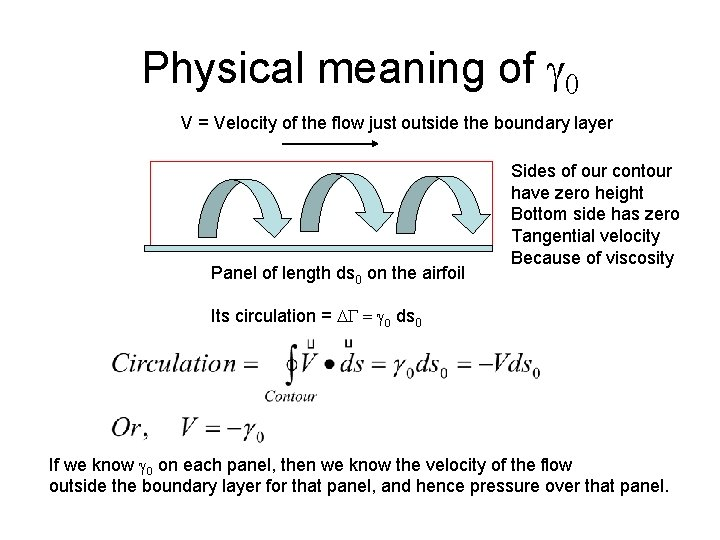Physical meaning of g 0 V = Velocity of the flow just outside the boundary layer Panel of length ds 0 on the airfoil Sides of our contour have zero height Bottom side has zero Tangential velocity Because of viscosity Its circulation = DG = g 0 ds 0 If we know g 0 on each panel, then we know the velocity of the flow outside the boundary layer for that panel, and hence pressure over that panel.Pressure distribution and Loads Since V = -g 0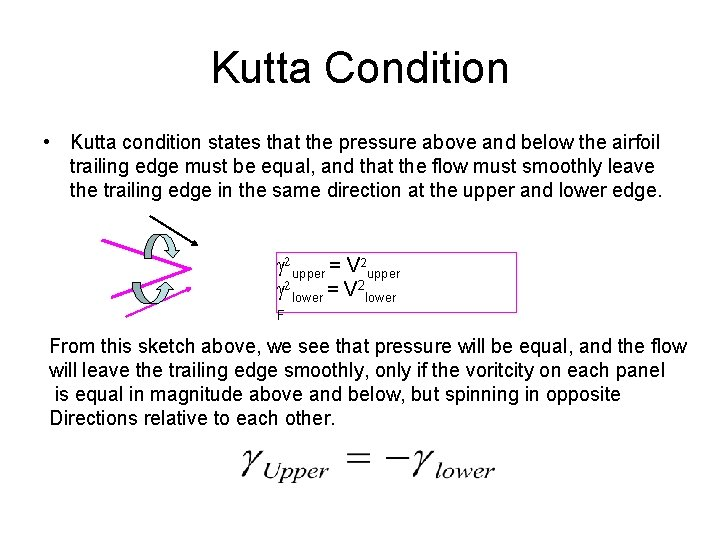Kutta Condition • Kutta condition states that the pressure above and below the airfoil trailing edge must be equal, and that the flow must smoothly leave the trailing edge in the same direction at the upper and lower edge. g 2 upper = V 2 upper g 2 lower = V 2 lower F From this sketch above, we see that pressure will be equal, and the flow will leave the trailing edge smoothly, only if the voritcity on each panel is equal in magnitude above and below, but spinning in opposite Directions relative to each other.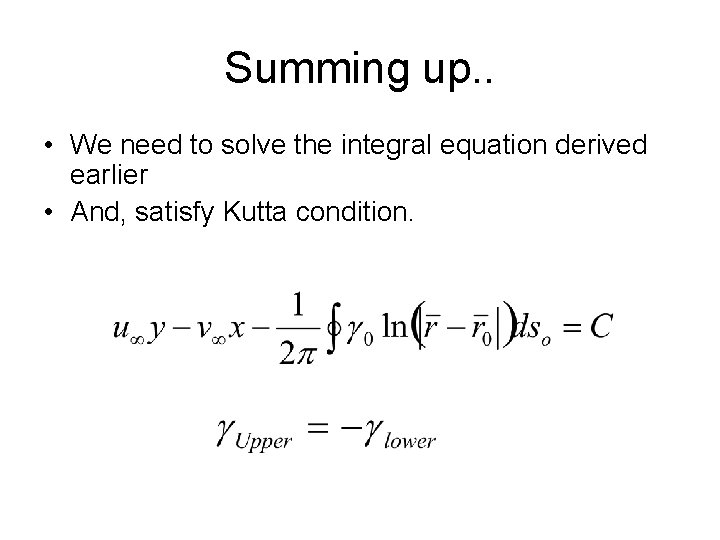Summing up. . • We need to solve the integral equation derived earlier • And, satisfy Kutta condition.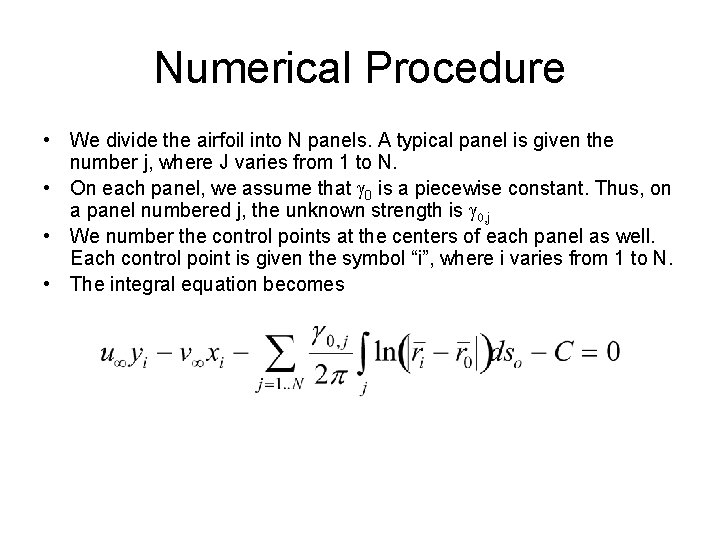Numerical Procedure • We divide the airfoil into N panels. A typical panel is given the number j, where J varies from 1 to N. • On each panel, we assume that g 0 is a piecewise constant. Thus, on a panel numbered j, the unknown strength is g 0, j • We number the control points at the centers of each panel as well. Each control point is given the symbol “i”, where i varies from 1 to N. • The integral equation becomes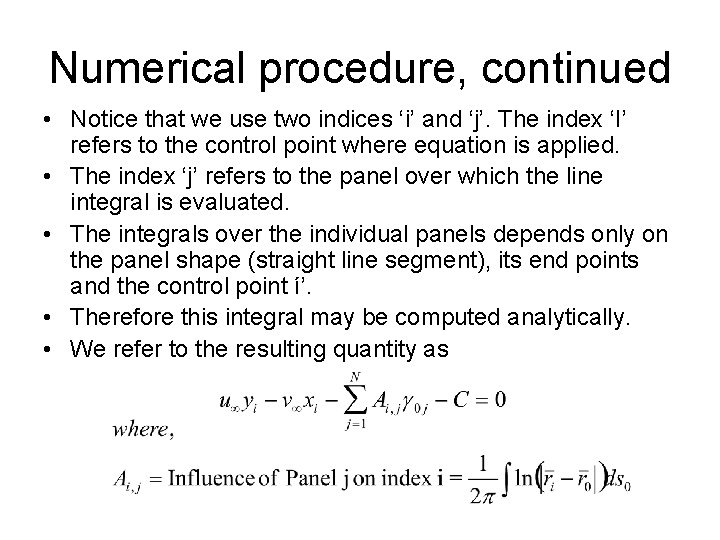Numerical procedure, continued • Notice that we use two indices ‘i’ and ‘j’. The index ‘I’ refers to the control point where equation is applied. • The index ‘j’ refers to the panel over which the line integral is evaluated. • The integrals over the individual panels depends only on the panel shape (straight line segment), its end points and the control point í’. • Therefore this integral may be computed analytically. • We refer to the resulting quantity as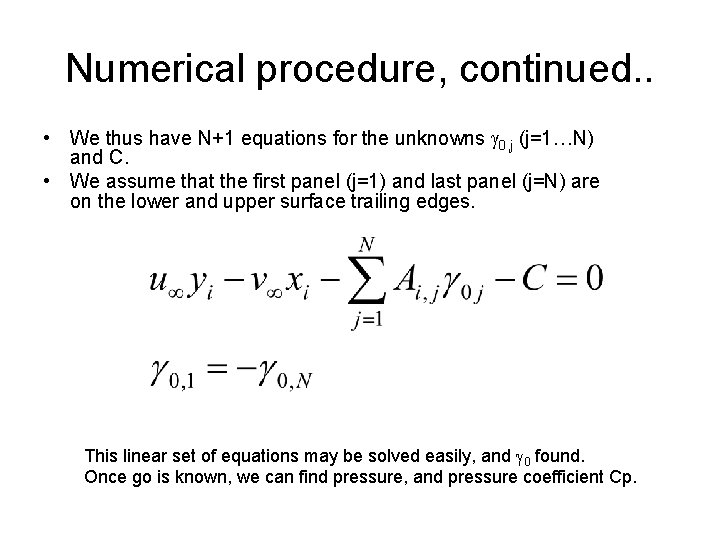Numerical procedure, continued. . • We thus have N+1 equations for the unknowns g 0, j (j=1…N) and C. • We assume that the first panel (j=1) and last panel (j=N) are on the lower and upper surface trailing edges. This linear set of equations may be solved easily, and g 0 found. Once go is known, we can find pressure, and pressure coefficient Cp.Panel code • Our web site contains a Matlab code I have written, if you wish to see how to program this approach in Matlab. • See http: //www. ae. gatech. edu/people/lsankar/AE 3903/Panel. m • And, sample input file http: //www. ae. gatech. edu/people/lsankar/AE 3903/panel. data. txt • An annotated file telling you what the avrious numbers in the input means is found at – http: //www. ae. gatech. edu/people/lsankar/AE 3903/Panel. Code. In put. txt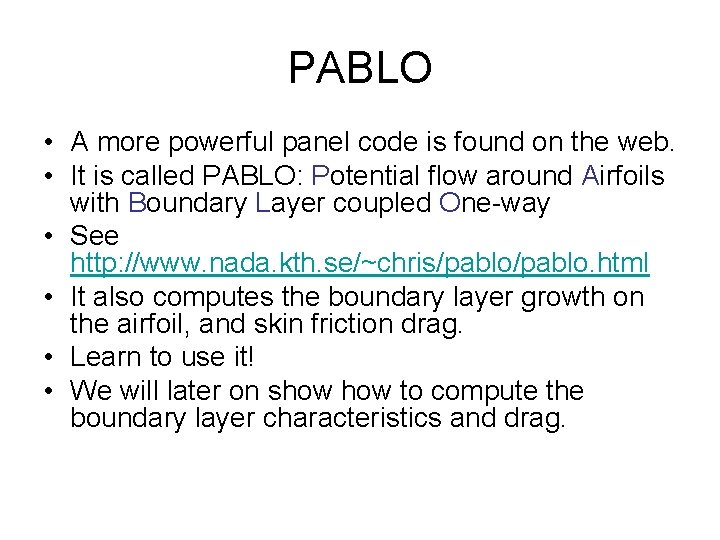PABLO • A more powerful panel code is found on the web. • It is called PABLO: Potential flow around Airfoils with Boundary Layer coupled One-way • See http: //www. nada. kth. se/~chris/pablo. html • It also computes the boundary layer growth on the airfoil, and skin friction drag. • Learn to use it! • We will later on show to compute the boundary layer characteristics and drag.